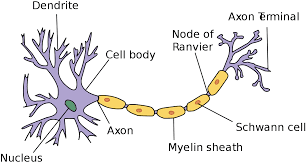## Posts

Showing posts from October, 2018

### Neural Network: Part 2Neural Network: Logistic Regression In this post, I'll deal with logic regression and its implementation of a single neuron network. I hope you have read my previous post on mattresses and its operation using NumPy package. You can click here to go to my previous post
Logistic regression basically computes the probability of the output to be one. For example, if we train our Logistic model to recognize the image of a dog then for any new image the model basically try to calculate the probability of whether the new image is a dog or not. the higher the probability will imply that the given image is of a dog.
A neuron is the primary and fundamental unit of computation for any neural network. A neuron will receive a vector that will include the input features. Each of the features will get multiplied with their corresponding weights and then a bias will be added to each of the features after which the weighted sum will be calculated. This will produce a linear output from the prev…

### Neural Network: Part 1Basics of Neurons
Hey everyone, long time no see. I have been working on some stuff so was busy and away from my blog. Well, as for my interest in AI, I have been working on neural networks and its implementation which I'll discuss in this post.

In this post, you will learn:
The basic concepts used in building a neural networkImplementing logistic regressionSingle Neural NetworkForward propagation and Backward propagationBuilding a small neural network using TensorFlowBuilding a deep neural network using TensorFlow. As we know, we humans learn from our experiences and through training. Our brain runs a very high speed and processes any activity quickly. Well, it actually depends on the type of activity, the person is working on. But as we all know, humans have a high grasping power and easily learn, understand, classify, remember and do various other things which machines take a hell lot of time to compute. All these computations are processed by our mighty brain. So to understand …